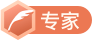# Anaconda3+PyCharm+Cuda10.1+Cudnn7.6+tensorflow2.2安装与配置教程

## 一、安装Anaconda3Anaconda3+tensorflow2.0.0+PyCharm安装与环境搭建

## 二、安装PyCharm## 三、安装TensorFlow2.2

### 3.1 创建虚拟环境

1）首先，从开始菜单找到并打开Anaconda Prompt。

2）然后，输入以下命令，新建一个虚拟环境

conda create -n tf2.2 python==3.7


3）等创建完毕后，进入虚拟环境

conda activate tf2.2


### 3.2 安装TensorFlow2.2

pip install -i https://pypi.douban.com/simple tensorflow==2.2.0### 3.3 检查是否安装成功

1）打开PyCharm，新建一个工程 First_Project2）配置虚拟环境3）选择合适的解释器，然后点击OK4）进入刚才新建的工程First_Project，新建一个python脚本mian.py，输入：

import tensorflow as tf
print(tf.__version__)## 四、安装nvidia驱动## 五、安装Cuda10.1### 5.1.查看电脑已装的cuda版本

Win+R—输入cmd—输入：nvcc --version### 5.2 安装Cuda## 六、安装Cudnn7.6## 七、检查安装与配置是否成功

import tensorflow as tf  # 导入TF库
from tensorflow.keras import layers, optimizers, datasets, Sequential, metrics  # 导入TF子库

# 1.数据集准备
(x, y), (x_val, y_val) = datasets.mnist.load_data()  # 加载数据集，返回的是两个元组，分别表示训练集和测试集
x = tf.convert_to_tensor(x, dtype=tf.float32)/255.  # 转换为张量，并缩放到0~1
y = tf.convert_to_tensor(y, dtype=tf.int32)  # 转换为张量（标签）
print(x.shape, y.shape)

train_dataset = tf.data.Dataset.from_tensor_slices((x, y))  # 构建数据集对象
train_dataset = train_dataset.batch(32).repeat(10)  # 设置批量训练的batch为32，要将训练集重复训练10遍

# 2.网络搭建
network = Sequential([
layers.Dense(256, activation='relu'),  # 第一层
layers.Dense(128, activation='relu'),  # 第二层
layers.Dense(10)  # 输出层
])
network.build(input_shape=(None, 28*28))  # 输入
# network.summary()

# 3.模型训练（计算梯度，迭代更新网络参数）
optimizer = optimizers.SGD(lr=0.01)  # 声明采用批量随机梯度下降方法，学习率=0.01
acc_meter = metrics.Accuracy()  # 创建准确度测量器
for step, (x, y) in enumerate(train_dataset):  # 一次输入batch组数据进行训练
with tf.GradientTape() as tape:  # 构建梯度记录环境
x = tf.reshape(x, (-1, 28*28))  # 将输入拉直，[b,28,28]->[b,784]
out = network(x)  # 输出[b, 10]
y_onehot = tf.one_hot(y, depth=10)  # one-hot编码
loss = tf.square(out - y_onehot)
loss = tf.reduce_sum(loss)/32  # 定义均方差损失函数，注意此处的32对应为batch的大小
acc_meter.update_state(tf.argmax(out, axis=1), y)  # 比较预测值与标签，并计算精确度
if step % 200 == 0:  # 每200个step，打印一次结果
print('Step', step, ': Loss is: ', float(loss), ' Accuracy: ', acc_meter.result().numpy())
acc_meter.reset_states()  # 每一个step后准确度清零AI 菌CSDN认证博客专家 CSDN博客专家 CSDN签约作者 算法实习生

11-085688
09-20233105-295585
11-1561
07-053240
07-31394
01-10851
03-07666
05-07331
07-29119点击重新获取扫码支付余额充值